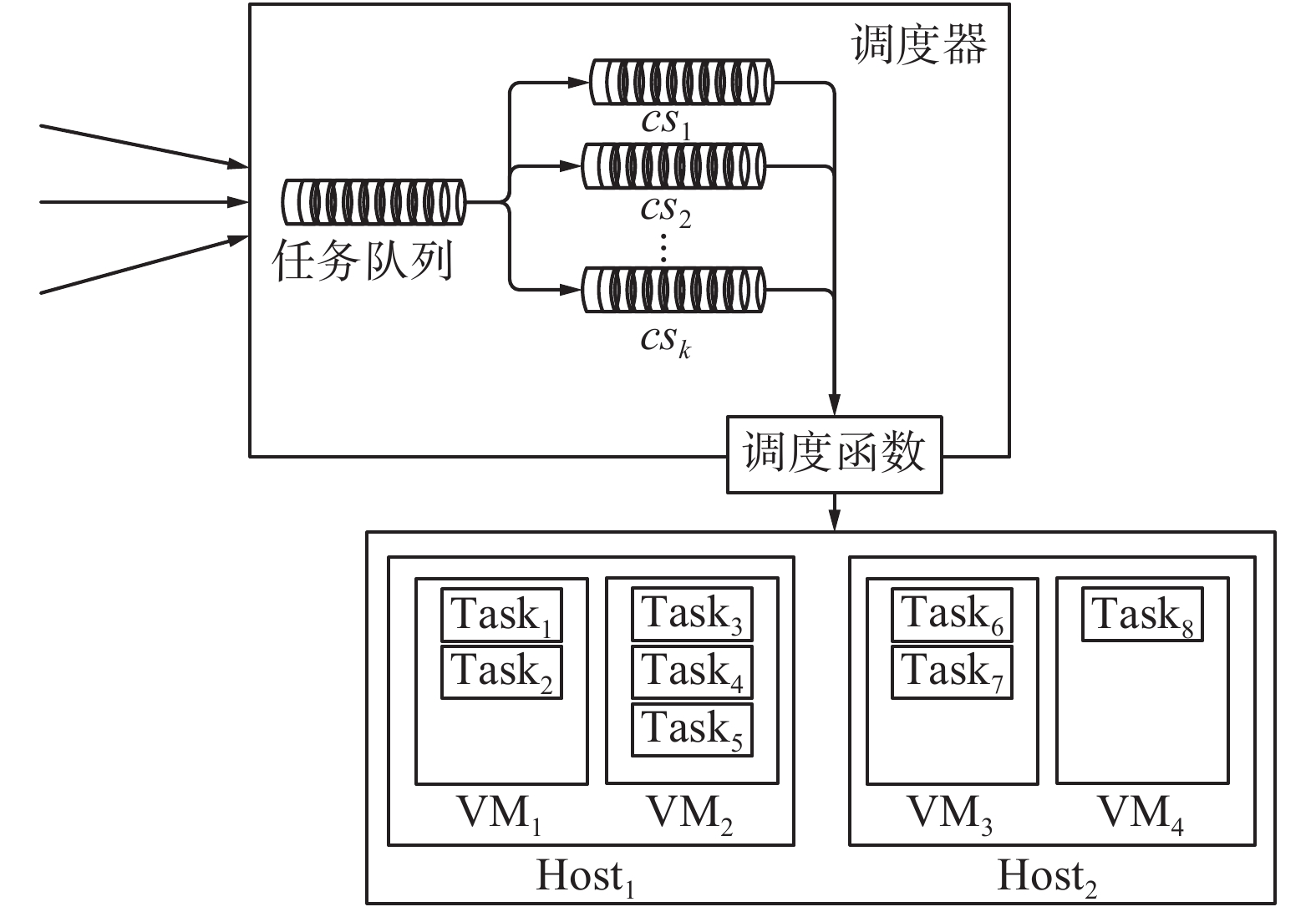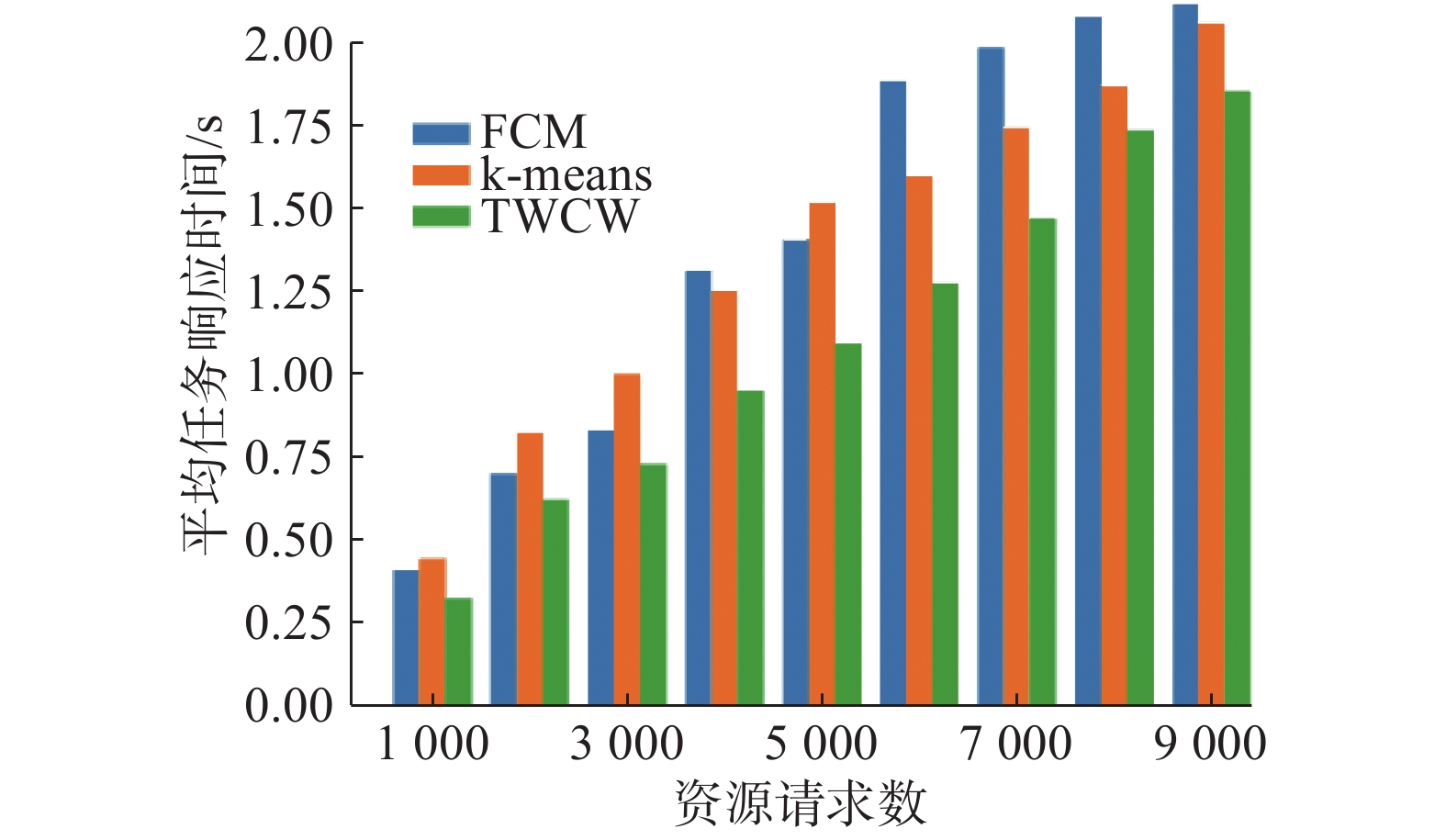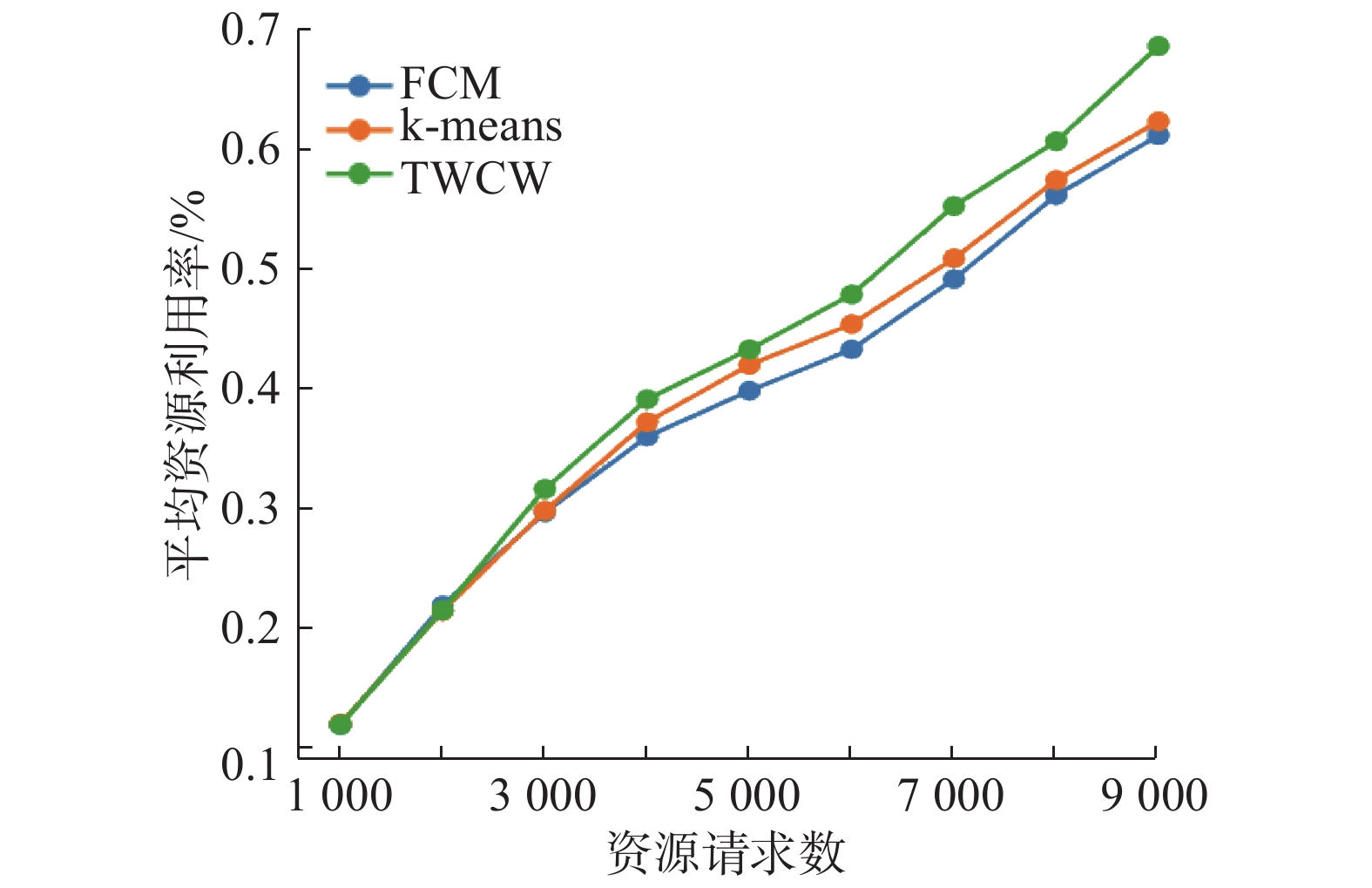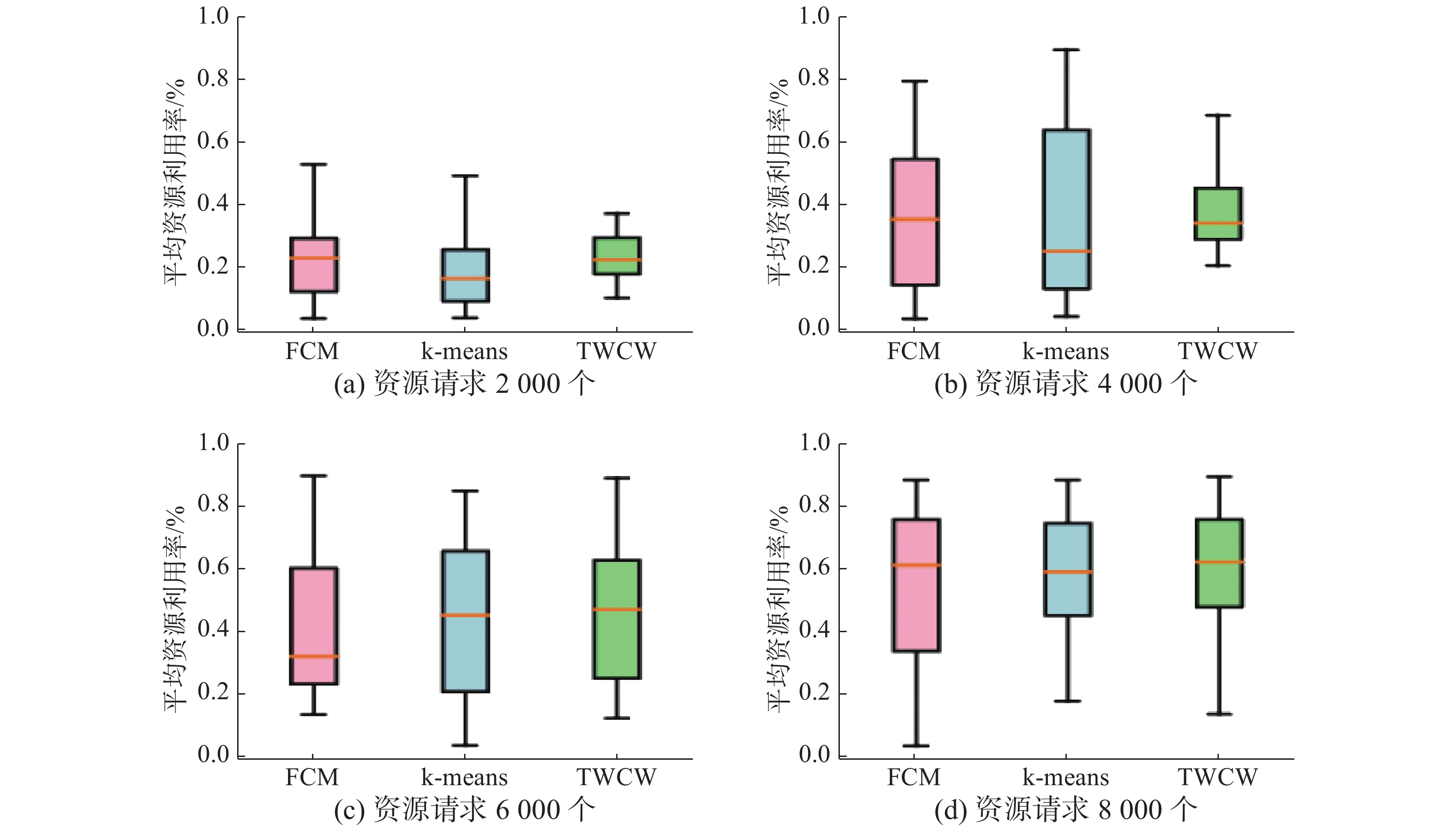﻿ 负载敏感的云任务三支聚类评分调度研究
«上一篇文章快速检索 高级检索

 智能系统学报2019, Vol. 14Issue (2): 316-322  DOI: 10.11992/tis.2017100040

### 引用本文WU Junwei, JIANG Chunmao. Load-aware score scheduling of three-way clustering for cloud task[J]. CAAI Transactions on Intelligent Systems, 2019, 14(2): 316-322. DOI: 10.11992/tis.201710004.### 文章历史

WU Junwei, JIANG Chunmao
School of Computer Science Technology and Information Engineering, Harbin Normal University, Harbin 150025, China
Abstract: A commercialized model is established for multi-objective optimization scheduling of service quality, balanced load, and economic principles in cloud computing. A three-way clustering weight (TWCW) algorithm is proposed to solve the problem of the low utilization rate of cluster resources. First, the diversified requirements of cloud tasks and the dynamic characteristics of resources are analyzed to cluster and divide the task set by the TWCW algorithm and then score scheduling by combination with task attributes. Simulation results based on Cloudsim show that compared with k-means and FCM clustering scheduling, the TWCW algorithm has significant improvements in the average task response time and resource utilization rate.
Key words: cloud computing    optimal scheduling    diversified requirement    dynamic resource    three-way clustering    scoring scheduling    response time of task    resource utilization rate

1 相关工作

 ${c_{(\alpha ,\beta )}}:\tau :U \to \{ {S_1},{S_2},{S_3}\}$ (1)

2 TWCW算法 2.1 任务模型

2.2 调度模型Download: 图 1 集群架构图 Fig. 1 Cluster architecture

1)调度器(Scheduler)：聚类调度，为任务选择合适节点。

2)计算节点(Host)：物理机节点，承载虚机。

3)虚机(VM)：资源分配的基本单元，执行请求任务。

2.3 算法描述

2.3.1 聚类

 $c{s_i} = (L(c{s_i}),M(c{s_i}))$ (2)

 $T = L(c{s_i}) \cup M(c{s_i}) \cup R(c{s_i})$ (3)
 $\begin{gathered} L(c{s_i}) \cap M(c{s_i}) = \text{Ø} \\ L(c{s_i}) \cap R(c{s_i}) = \text{Ø} \\ M(c{s_i}) \cap R(c{s_i}) = \text{Ø} \\ \end{gathered}$ (4)

1) 寻找最佳聚类数，使用聚类算法(如k-means)聚类任务集合 $T$ ，根据类簇间离散度与类簇内聚合度择优确定最佳聚类结果；

2) 确定类簇域对象，首先通过近邻域确定类簇 $M$ 域对象，然后使用差值排序进一步划分类簇 $L$ 域与 $M$ 域对象，其具体内容如下：对于类簇 $c{s_k}$ ，任务 ${t_i}$ ${t_j}$ 与近邻域 ${\rm Nei{g}_q}({t_i})$ ，其中，类簇 $c{s_k}$ 存在中心点 ${\rm centroi{d}_k}$ ${\rm Nei{g}_q}({t_i})$ 表示在欧式距离上离 ${t_i}$ 最近的 $q$ 个任务，当 ${t_i} \notin c{s_h}$ ${t_j} \in Nei{g_q}({t_i})$ 时，若 ${t_j} \in c{s_h}$ ，则确定 ${t_i}$ $M(c{s_h})$ 对象，然后根据类簇 $c{s_h}$ 内任务集合 ${t_1},{t_2}, \cdots ,{t_l}$ 与中心点 ${\rm centroi{d}_h}$ 的欧式距离差值排序，确定差值最大的任务对 ${t_{p - 1}}$ ${t_p}$ ，将 ${t_1},{t_2}, \cdots ,{t_{p - 1}}$ 划分到 $L(c{s_h})$ 中， ${t_p},{t_{p + 1}}, \cdots ,{t_l}$ 划分给 $M(c{s_h})$ 。其算法描述如下：

1) 初始化， $k = 2$

2) 随机选取 $k$ 个聚类中心 ${v_1},{v_2}, \cdots ,{v_k}$

3) 对于每个任务 ${t_i}$ ，计算其到每个聚类中心 ${v_i}$ 的距离，将其划分到距离最小的类中；

4) 更新聚类中心 $v = \{ v_1', v_2',\cdots ,v_k'\}$ ;

5) 如果聚类中心不发生变化至6)；否则转至3)；

6) 计算 ${\rm CVIN}(CS)$ ，如果 $k \leqslant \sqrt N$ ,那么 $k = k + 1$ ，转至2)；否则转至7)；

7) 考查任务 ${t_i}$ 和类 $cs$ ，其中 ${t_i} \in cs,{t_j} \in {\rm Nei{g}_q}(t)$ 。如果 ${t_j} \in cs$ ，那么把 ${t_i}$ 划分到 $M(cs)$

8) 对于类中剩余非 $M$ 域中对象，根据差值排序法，找到第一个距离差值最大的对象对 ${t_{i - 1}}$ ${t_i}$ ，把 ${t_i}$ 及其后的对象划分到 $M(cs)$

9) 算法结束，输出结果 $CS = \{ (L(c{s_1}),M(c{s_1})), \cdots ,$ $(L(c{s_k}),M(c{s_k}))\}$

2.3.2 评分

 ${{ W}_{N{\text ×}M}} = \left[ {\begin{array}{*{20}{c}} {{w_{1,1}}}&{{w_{1,2}}}& \cdots &{{w_{1,M}}} \\ {{w_{2,1}}}&{{w_{2,2}}}& \cdots &{{w_{2,M}}} \\ \vdots & \vdots &{}& \vdots \\ {{w_{N,1}}}&{{w_{N,2}}}& \cdots &{{w_{N,M}}} \end{array}} \right],\;N = 3,M = k$ (5)

 ${{ W}_{3 \times 4}} = \left[ {\begin{array}{*{20}{c}} 4&3&2&1 \\ 3&1&4&2 \\ 2&4&1&3 \end{array}} \right]$

2.3.3 调度

 ${{\rm spac{e}}_{i,j}} = ({{{{\rm allocabl{e}}_{i,j}} - {{\rm allocate{d}}_{i,j}})}/{{{\rm allocabl{e}}_{i,j}}}}$ (6)

 ${S_i} = \sum\limits_{j = 1}^N {{\mu _j} \cdot {{\rm spac{e}}_{i,j}},\;N = 3}$ (7)

TWCW算法可最大限度的利用空闲资源，根据任务属性评估节点列表 $H$ 的剩余可分配空间 ${S_i}$ ，选择资源可分配空间最大的主机作为目标节点，如式(8)所示。

 ${h_{\rm{target}}} = \max (\sum\limits_{j = 1}^N {{\mu _j}{\rm spac{e}}}_{i,j} ),\;N = 3,i = 1,2, \cdots ,m$ (8)

TWCW算法可最大限度的利用空闲资源，根据任务属性评估节点列表 $H$ 的剩余可分配空间 ${S_i}$ ，选择资源可分配空间最大的主机作为目标节点，如式(8)所示。

 ${\mu _j} = \frac{{{w_{j,i}}}}{{\displaystyle\sum\limits_{j = 1}^N {{w_{j,i}}} }},N = 3$ (9)

1) 初始化权重因子 $\mu$

2) 获取集群资源resource，初始化迭代变量i=0；

3) 计算在第 $i$ 维属性中，任务 $t$ 在资源resource中的比重propi=t.attri/resourcei

4) 如果 $i < N$ $i = i + 1$ ，转至步骤3)。否则转至5)；

5) 确定propi中比重最大的第 $l$ 维属性，将 ${\mu _l}$ 置为1，算法结束。

2.3.4 时间复杂度分析。

TWCW算法包括了任务聚类、评分与调度，其中：

1) 三支聚类时间复杂度分析。设任务集合的任务数为 $n$ ，聚类分支数为 $k$ ，近邻数为 $q$ ，k- ${\rm means}$ 的迭代次数为 $I$ ，寻找最佳聚类数目的时间复杂度为 $O({n^2}I + {n^2}q)$ ，确定分支域对象的时间复杂度为 $O(n\log n + knq)$ 

2) 类簇评分时间复杂度分析。设聚类分支数为 $k$ ，确定类簇中心在单个属性的排序算法的时间复杂度为 $O(k\log k)$

3) 选择目标主机时间复杂度分析。设任务集合的任务数为 $n$ ，主机列表的节点数为 $m$ ，对于单个任务选择目标节点的时间复杂度为 $O(m)$ ，那么选择目标主机的时间复杂度为 $O(nm)$

3 实验及数据分析 3.1 实验环境与参数设置表 1 任务参数 Tab.1 Task parameters setup

3.2 任务平均响应时间比较实验Download: 图 2 资源请求增加时响应时间对比 Fig. 2 The average response time comparison when requests are increased

3.3 集群资源利用率比较实验Download: 图 3 资源请求增加时平均利用率对比 Fig. 3 The resource average utilization comparison when requests are increasedDownload: 图 4 资源请求增加时资源使用情况对比 Fig. 4 The resource usage comparison when requests are increased

4 结束语

  BOHRER P, ELNOZAHY E N, KELLER T, et al. The case for power management in web servers[M]//GRAYBILL R, MELHEM R. Power Aware Computing. Boston, MA, USA: Springer, 2002: 261–289. (0)  BARROSO L A, HÖLZLE U. The case for energy-proportional computing[J]. Computer, 2007, 40(12): 33-37. DOI:10.1109/MC.2007.443 (0)  REISS C, TUMANOV A, GANGER G R, et al. Heterogeneity and dynamicity of clouds at scale: Google trace analysis[C]//Proceedings of the third ACM Symposium on Cloud Computing. New York, NY, USA, 2012: 1–13. (0)  LIU Zitao, CHO S. Characterizing machines and workloads on a Google cluster[C]//Proceedings of 2012 41st International Conference on Parallel Processing Workshops. Pittsburgh, PA, USA, 2012: 397–403. (0)  左利云. 云计算中基于任务特性和资源约束的调度方法研究[D]. 广州: 华南理工大学, 2016: 1–139. ZUO Liyun. The scheduling methods based on the task features and resource constraints in cloud computing[D]. Guangzhou, China: South China University of Technology, 2016: 1–139. (0)  刘家志. 模糊C-均值算法在任务调度问题上的应用[C]//第十届中国通信学会学术年会论文集. 沈阳, 中国, 2014: 310–313. LIU Jiazhi. Application of fuzzy c-means algorithm in task scheduling problem[C]//A Collection of Academic Annual Meetings of the Tenth China Communications Society. Shenyang, China, 2014: 310-313. (0)  封良良, 夏晓燕, 贾振红, 等. 实验基于资源预先分类的云计算任务调度算法[J]. 计算机仿真, 2013, 30(10): 363-367, 410. FENG Liangliang, XIA Xiaoyan, JIA Zhenhong, et al. Task scheduling algorithm based on improved particle swarm optimization algorithm in cloud computing environment[J]. Computer simulation, 2013, 30(10): 363-367, 410. DOI:10.3969/j.issn.1006-9348.2013.10.083 (0)  张以利, 杨万扣. 云环境下基于任务分类和LPM优化模型的调度算法[J]. 微型电脑应用, 2013, 29(10): 5-8. ZHANG Yili, YANG Wankou. Scheduling algorithm based on task classifying and linear pogramming model in a cloud environment[J]. Microcomputer application, 2013, 29(10): 5-8. DOI:10.3969/j.issn.1007-757X.2013.10.002 (0)  陈晶晶. 云环境下基于非均匀粒度分类的任务调度算法研究[D].南京: 南京邮电大学, 2015: 1–59. CHEN Jingjing. Research on task scheduling algorithm based on non-uniform granularity classification in cloud environment[D]. Nanjing, China: Nanjing University of Posts and Telecommunications, 2015: 1–59. (0)  高正九, 郑烇, 辛波, 等. 基于任务分类的延迟调度算法[J]. 计算机系统应用, 2014, 23(9): 139-143. GAO Zhengjiu, ZHENG Quan, XIN Bo, et al. Delay scheduling algorithm based on task classification[J]. Computer systemsand & applications, 2014, 23(9): 139-143. DOI:10.3969/j.issn.1003-3254.2014.09.026 (0)  YAO Yiyu. The superiority of three-way decisions in probabilistic rough set models[J]. Information sciences, 2011, 181(6): 1080-1096. DOI:10.1016/j.ins.2010.11.019 (0)  YAO Yiyu. Three-way decisions with probabilistic rough sets[J]. Information sciences, 2010, 180(3): 341-353. DOI:10.1016/j.ins.2009.09.021 (0)  YAO Yiyu. Three-way decision: an interpretation of rules in rough set theory[C]//Proceeding of the 4th International Conference Rough Sets and Knowledge Technology. Gold Coast, Australia, 2009: 642–649. (0)  ZHOU Bing, YAO Yiyu, LUO Jigang. Cost-sensitive three-way email spam filtering[J]. Journal of intelligent information systems, 2014, 42(1): 19-45. DOI:10.1007/s10844-013-0254-7 (0)  ZHANG Hengru, MIN Fan, HE Xu, et al. A hybrid recommender system based on user-recommender interaction[J]. Mathematical problems in engineering, 2015, 2015: 145636. (0)  YAO Jingtao, AZAM Nouman. Web-based medical decision support systems for three-way medical decision making with game-theoretic rough sets[J]. IEEE transactions on fuzzy systems, 2015, 23(1): 3-15. DOI:10.1109/TFUZZ.2014.2360548 (0)  QIAN Yuhua, ZHANG Hu, SANG Yanli, et al. Multigranulation decision-theoretic rough sets[J]. International journal of approximate reasoning, 2014, 55(1): 225-237. DOI:10.1016/j.ijar.2013.03.004 (0)  LI Huaxiong, ZHOU Xianzhong, HUANG Bing, et al. Cost-sensitive three-way decision: a sequential strategy[M]//LINGRAS P, WOLSKI M, CORNELIS C, et al. Rough Sets and Knowledge Technology. Berlin, Germany: Springer, 2013: 325–337. (0)  LIU Dun, LI Tianrui, LIANG Decui. Three-way decisions in dynamic decision-theoretic rough sets[M]//LINGRAS P, WOLSKI M, CORNELIS C, et al. Rough Sets and Knowledge Technology. Berlin, Germany: Springer, 2013: 291–301. (0)  SHE Yanhong. On determination of thresholds in three-way approximation of many-valued nm-logic[M]//CORNELIS C, KRYSZKIEWICZ M, ŚLȨZAK D, et al. Rough sets and Current Trends in Computing. Cham, Germany: Springer, 2014: 136–143. (0)  于洪. 三支聚类分析[J]. 数码设计, 2016, 5(1): 31-35, 30. YU Hong. Three-way cluster analysis[J]. Peak data science, 2016, 5(1): 31-35, 30. (0)  于洪, 毛传凯. 基于k-means的自动三支决策聚类方法[J]. 计算机应用, 2016, 36(8): 2061-2065, 2091. YU Hong, MAO Chuankai. Automatic three-way decision clustering algorithm based on k-means[J]. Journal of computer applications, 2016, 36(8): 2061-2065, 2091. (0)